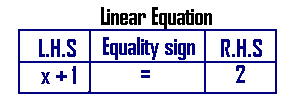## Linear Equation in One Variable

#### Basic Algebra > Linear Equation in One Variable

Linear Equation in One Variable

1. Definition of an Equation:

What is an equation?
A statement of equality of two algebraic expressions in or more variables is called an equation.

Examples: 1. x + 1 = 2 and
2. 2y + 3 = 5

In 1 above, x + 1 is an algebraic expression in the variable x. we read it as “ x plus 1 is equal to 2”.
x is some number, called variable.

In 2 above, 2y + 3 is an algebraic expression in the variable y, we read it as “ 2 times y or 2y plus 3 is equal to 5”. y is some number, called variable

2. The three components of an equation

Every equation has a Left hand side, the equality sign ‘=’ and the Right hand side. the three components in the equation x + 1 = 2 are :L.H.S = Left Hand Side
R.H.S = Right Hand Side

3. Solution/Root of an Equation:

the value of x, i.e. some number for x, which makes the equation a true statement is called solution or root of the equation.

In simple words, if the L.H.S. and R.H.S become equal for some number plugged in for x, then the number, also called value, is the solution or root of the equation.

In x + 1 = 2, what should be plugged in for x so that L.H.S. becomes equal to R.H.S?

It is 1, i.e. if 1 is plugged in for x, the two sides become equal.

This number or value 1 for x is called root or solution of the equation. Transposition Rule:

Transposition Rule

1. The transposition rule applies on addition and subtraction.

Transposition Rule
Terms can be transposed (shifted) between either sides of the equality symbol “=” with a change in sign of the transposed terms”

Example: x + 1 = 2.
1 can be transposed to right side by inversing its sign
i.e. x = -1 + 2 so that we have x = 1
transposition conforms with the rule:

“same numbers can be added on both sides of an equation”

So, in x + 1 = 2, to find x, we need to get rid of 1 on the left hand side, to do this add -1 on both sides of the equation.
x +1-1 = -1 + 2,
x = 1

Application of Linear Equations or Word Problems on Linear Equations in one Variable

Problem 1:
The sum of two consecutive numbers is 25. Find the numbers.

Solution:
Let the two consecutive numbers be x andx+1.
So we can set up the following linear equation:
Given that x + x+1= 25,
2x = 24, {from transposition rule, inverse sign of 1 on taking it to right side}
x = 24/2 = 12, {multiplication changes to division}
So the other number is x + 1 = 12 + 1 = 13
Therefore, the two numbers are 12 and 13

Problem 2:
John is 15 years now. He is 10 years older than his brother Tom. How old is Tom 10 years from now?

Solution: Let Tom be x years old now.
So, we set up the linear equation:
x + 10 = 15, x = 5. {from transposition rule, inverse sign of 10 on taking it to right side}
So, tom is 5 years old now.
10 years from now, tom will be 10 + 5 = 15

Problem 3:
20 years from now, Nancy will become three times as old as he is now. Find her age now.

Solution:
Let Nancy be x years old now.
20 years from now, she will be x +20
But 20 years from now, she we thrice her present age, x i.e. 3x
So, x + 20 = 3x, or 3x = x + 20,
3x – x = 20, {from transposition rule}
2x = 20,
x = 20/2 = 10